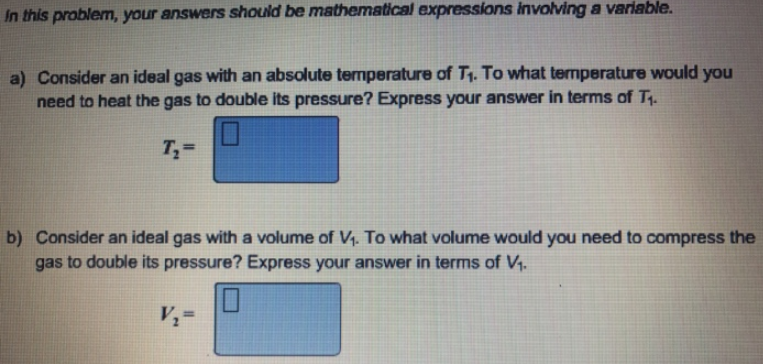Chemistry Practice Problems Chemistry Gas Laws Practice Problems Solution: In this problem, your answers mathematical express...

# Solution: In this problem, your answers mathematical expressions involving a variable. a) Consider an ideal gas with an absolute temperature of T1. To what temperature would you need to heat the gas to double its pressure? Express your answer in terms of T1. b) Consider an ideal gas with a volume of V1. To what volume would you need to compress the gas to double its pressure? Express your answer in terms of V1.

###### Problem

a) Consider an ideal gas with an absolute temperature of T1. To what temperature would you need to heat the gas to double its pressure? Express your answer in terms of T1

b) Consider an ideal gas with a volume of V1. To what volume would you need to compress the gas to double its pressure? Express your answer in terms of V1.View Complete Written Solution

Chemistry Gas Laws

Chemistry Gas Laws

#### Q. A sample of an ideal gas has a volume or 2.25 L at 284 K and 1.13 atm. Calculate the pressure when the volume is 1.83 L and the temperature is 298 K.

Solved • Fri Oct 05 2018 14:27:18 GMT-0400 (EDT)

Chemistry Gas Laws

#### Q. A sample of an ideal gas has a volume or 2 25 L at 284 K and 1.13 atm. Calculate the pressure when the volume is 1.83 L and the temperature is 298 K

Solved • Mon Sep 17 2018 16:59:14 GMT-0400 (EDT)

Chemistry Gas Laws

#### Q. A sample of neon gas at 305 K and 0.372 atm occupies a volume of 1.89 L. If the pressure of the gas is increased, while at the same time it is heated ...

Solved • Wed Sep 12 2018 11:38:19 GMT-0400 (EDT)

Chemistry Gas Laws

#### Q. An ideal gas is allowed to expand from 9.00 L to 76.5 L at constant temperature. By what factor does the volume increase? The pressure will           ...

Solved • Wed Sep 12 2018 10:46:01 GMT-0400 (EDT)